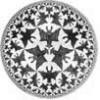#### You may also like### How Many Geometries Are There?

An account of how axioms underpin geometry and how by changing one axiom we get an entirely different geometry.### When the Angles of a Triangle Don't Add up to 180 Degrees

This article outlines the underlying axioms of spherical geometry giving a simple proof that the sum of the angles of a triangle on the surface of a unit sphere is equal to pi plus the area of the triangle.### Flight Path

Use simple trigonometry to calculate the distance along the flight path from London to Sydney.

# Over the Pole

##### Age 16 to 18Challenge Level

Knowing the angle of latitude you can work out the radius of the circle of latitude and half its circumference. You can also work out the arc length of the route over the pole.

It would be a good idea to draw a graph for the ratio of these distances for different angles of latitude.

Can you explain why this ratio tends to a limit?